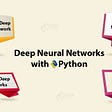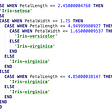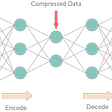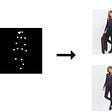# Coding Multi-Agent Reinforcement Learning algorithms

Multi-Agent learning involves two strategies. Concurrent and centralized. In concurrent learning, each agent has an actor, each learning multiple policies. In centralized learning, the actor is decentralized and the critic is centralized. The actor learns its policy and critic take the actions taken by all agents. Critic returns different reward structures for each agent. E.g., cooperative, competitive, mixed task

Actor Parameter sharing can be enabled (single policy for all actors) or disabled among the actors. Enable critic parameter sharing when all agents are homogeneous in the reward structure.

# MAA2C

The architecture of Actor-Critic networks

`actions = [Actor()] * n_agentsif concurrent:    critic = [Critic(state_dim,action_dim)] * n_agentsif centralized:    critic = [Critic(state_d*n_agents, actions_d*n_agents)]*n_agentsoptimizers = [Adam(actor) for actor in actors]if actor_parameter_sharing:    for agent in agents[1:]:         actor[agent] = actorif critic_parameter_sharing:    for agent in agents[1:]:         critic[agent] = critic`

Actions, Rewards, and Values

`# Actionsactions = [Actor(state[:,agent_id,:]),...]# Valuesif concurrent:    critics[agent_id](state[:,agent_id,:], action_var[:,agent_id,:])if centralized:    critics[agent_id](whole_state, whole_action)# Rewards (R)for agent_id in range(n_agents):  R[:,agent_id]=self.compute_reward(R[:,agent_id],critics[agent_id])`

Train

`for agent_id in range(n_agents):     # update actor network     actor_optimizers[agent_id].zero_grad()     actions = actors[agent_id](state[:,agent_id,:])     values = critics[agent_id](...) # concurrent; centralized     advantage = rewards_var[:,agent_id,:] - values     actor_loss = ...     actor_loss.backward()     actor_optimizers[agent_id].step()     # update critic network     critic_optimizers[agent_id].zero_grad()     target_values = rewards_var[:,agent_id,:]     critic_loss = ...     critic_loss.backward()     critic_optimizers[agent_id].step()`

`Ref: https://github.com/ChenglongChen/pytorch-madrl/blob/master/MAA2C.py`

`Agent()- Replay buffer    - PER    - UER- Epsilon decay of rewards- Define neural network modelMulti-Agent()- General structure   - get actions     for agent in agents: actions.append(agent.greedy_actor(state))   - get rewards (combined for all agents)   - store in replay buffer   - for agent in agents: agent.update_target_network()   - ... etc`

`actor_loss = ... + 1e-3 * regularization termminimize_and_clip(actor_loss)critic_loss = ... + 1e-3 * regularization termminimize_and_clip(critic_loss)`

make_env

`def make_env(scenario_name, arglist, benchmark=False):        from multiagent.environment import MultiAgentEnv        import multiagent.scenarios as scenarios    scenario = scenarios.load(scenario_name + ".py").Scenario()         world = scenario.make_world()    if benchmark:                 env = MultiAgentEnv(world, scenario.reset_world,                 scenario.reward, scenario.observation,                 scenario.benchmark_data)      else:                env = MultiAgentEnv(world, scenario.reset_world,                 scenario.reward, scenario.observation)        return env`

Train

`# Create environment        env = make_env(arglist.scenario, arglist, arglist.benchmark)  # Create agent trainers        obs_shape_n = [env.observation_space[i].shape for i in range(env.n)]trainers = [MADDPG(obs_shape_n[i]) for i in range(agents)]while True:   action_n=[agent.action(obs) for agent,obs in zip(trainers,obs_n)]   ..   env.step()   ..   for i, agent in enumerate(trainers):       agent.experience(obs_n[i], action_n[i], rew_n[i],                          new_obs_n[i], done_n[i], terminal)   # Collect rewards   # Save model`

# MA-PPO

It is similar to the multi-agent A2C implementation but with PPO.

# MA-SAC

SAC uses 1 Actor, 2 Critic networks (V valued and Q valued critics), and 1 target Critic Network thereby using the advantage of both Actor-Critic and DQN based solutions. Here we are using 2 agents.

`# Agent 0actor_0 = Actor(state_size,action_size).to(device)critic0_q0 = Critic_Q(state_size, action_size, self.seed).to(device)critic0_q1 = Critic_Q(state_size, action_size, self.seed).to(device)critic0_v = Critic_V(state_size, self.seed).to(device)critic0_target_v = Critic_V(state_size, self.seed).to(device)actor0_optimizer = optim.Adam(self.actor_0.parameters(),lr=lr,weight_decay=WEIGHTDECAY)critic0q0_optimizer = optim.Adam(self.critic0_q0.parameters(),lr=lr,weight_decay=WT_DECAY)critic0q1_optimizer = optim.Adam(self.critic0_q1.parameters(),lr=lr,weight_decay=WT_DECAY)critic0_v_optimizer = optim.Adam(self.critic_v.parameters(),lr=lr,weight_decay=WT_DECAY)# Agent 1actor_1 = Actor(state_size,action_size).to(device)critic1_q0 = Critic_Q(state_size, action_size, self.seed).to(device)critic1_q1 = Critic_Q(state_size, action_size, self.seed).to(device)critic1_v = Critic_V(state_size, self.seed).to(device)critic1_target_v = Critic_V(state_size, self.seed).to(device)actor1_optimizer = optim.Adam(self.actor_1.parameters(),lr=lr,weight_decay=WEIGHTDECAY)critic1q0_optimizer = optim.Adam(self.critic0_q0.parameters(),lr=lr,weight_decay=WT_DECAY)critic1q1_optimizer = optim.Adam(self.critic0_q1.parameters(),lr=lr,weight_decay=WT_DECAY)critic1_v_optimizer = optim.Adam(self.critic1_v.parameters(),lr=lr,weight_decay=WT_DECAY)# find loss# soft_update parametersdef soft_update(self, local_model, target_model, tau):    for target_param, local_param in            zip(target_model.parameters(), local_model.parameters()):        target_param.data.copy_( tau*local_param.data +                          (1.0-tau)*target_param.data)`

Train

`def Train(n_episodes=2000):    scores = []    scores_window = deque(maxlen=100)    for i_episode in range(1, n_episodes+1):        env_info = env.reset(train_mode = True)[brain_name]        state = env_info.vector_observations        score = np.asarray([0.,0.])        while True:            action = agent.act(state)            env_info = env.step(action)[brain_name]            next_state, reward, done = env_info.vector_observations,                                env_info.rewards,env_info.local_done            agent.step(state, action, reward, next_state, done)            state = next_state # update the state information            score += np.max(reward) # accumalate the reward            if np.any(done):                break                scores_window.append(np.max(score))         scores.append(np.max(score))         print(i_episode, np.mean(scores_window))        if i_episode % 100 == 0:            print(i_episode, np.mean(scores_window))        if np.mean(scores_window)>=0.5:            print('\nEnv solved in {:d} episodes!\tAverage Score: {:.2f}'.format(i_episode-100, np.mean(scores_window)))        # save checkpoints            break    return scoresscores = Train()`

# MA-TRPO

It is similar to the multi-agent A2C implementation but with TRPO.

--

--

--

## More from Amulya Reddy Konda

Consultant

Love podcasts or audiobooks? Learn on the go with our new app.

## Deep Neural Networks With Python## Decision trees and SQL (Part 2 — SQL as decision tree)## “Theory of Vibe”: A conversation with Peli Grietzer## Deep Image Prior## Bias-Variance Trade-Off in Machine Learning## How do we use NLP to improve our Tech Hiring?## Artificial Intelligence- Boon OR Bane?Consultant

## Build Your Own Reinforcement Learning Algorithm## Intuition behind learning in Reinforcement Learning